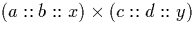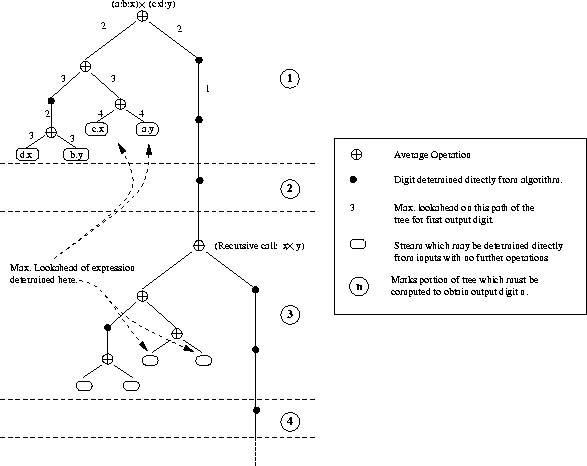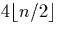Next: Division Up: Multiplication Previous: Dyadic Stream Multiplication

### Signed Binary Stream Multiplication

The signed binary stream multiplication algorithm (section 4.3.2) is more complex than the dyadic version. Although there is no possibility of the digits swelling, the lookahead properties are worse and the branching different. The operation of the algorithm used to computeis illustrated in figure 7.1.

The figure uses the fact that the average operation requires at most two digits of the input streams to compute one digit of output. The part of the tree which governs the lookahead is marked. To obtain one digit of output, a maximum of four digits is required from x and y, a total lookahead of six digits. Four two output digits, seven digits of the inputs are required. To obtain three output digits we also examine the recursive call. This requires a lookahead of six on the operands it was called with x and y, which is a total lookahead of eight. We can see that the maximum lookahead required for n digits is (n+5), and also that the minimum is (n+2) digits of the input streams. This range of possible lookaheads exists because the signed binary average algorithm may only require one digit of input to determine one digit of output in certain situations.The branching which will occur is also clear from the figure. The recursive call is made once for ever two digits of output. At each recursive call we introduce another four average operations. Hence obtaining n digits of output will result inaverage operations. In fact by optimising the algorithm to fix cases where the we are averaging streams with one input zero using `cons' operations, etc., this becomes an upper bound on the number of average operations.

It is unlikely that this is the most efficient algorithm with regards lookahead requirements as we could get an algorithm from signed binary to signed binary with a constant of lookahead (n+2) via the dyadic digits, albeit one which suffers from the problems of swelling dyadic digit sizes to some extent.Next: Division Up: Multiplication Previous: Dyadic Stream Multiplication
Martin Escardo
5/11/2000